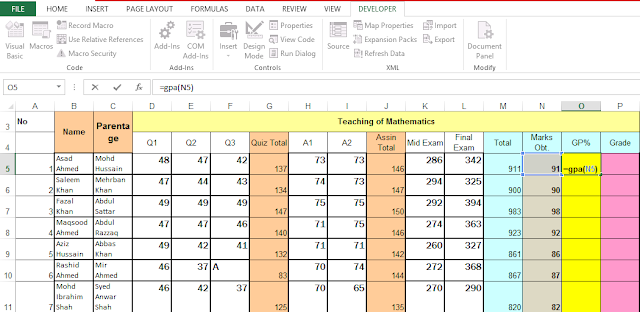# GPA Calculation

## GPA

GPA stands for Grade Point Average, this is a number that shows how well or how high you scored in your examination courses on average.

## How to Calculate GPA

We are going to Calculate GPA (grade point average) the usage of VBA Procedure in excel 2007-2016

## calculate GPA using vba in excel

To calculate GPA using vba in excel We need to calculate GPA (grade point average) with college students examination numbers and We have an excel sheet with students courses scores.

By starting the Microsoft Excell VisualBasic (VBA) Applications window you could get right of entry to the VisualBasic (VBA) surroundings in Excel 2007-2016

To begin with, ensure that the DEVELOPER tab is seen withinside the toolbar in Excel 2007.
The Excel 2007 DEVELOPER tab is the tool that has the buttons on toolbar to open the MS excel VisualBasic (VBA) editor.
To display the Developer tab, click on the FILE menu and then choose the button Options beginning from the drop menu.

At the factor while the Excel Options dialogue window suggests up, click on, after that click on at the Customize Ribbon at the left side.

Click from the dialogue window Excel Options at the VBA Developer checkbox below the listing of MainTabs at the right. Then click on at the OK button.From the toolbar at the top of the screen Select the VBA DEVELOPER tab. Then click on the Visual Basic option withinside the Code group.

Presently the Microsoft Excel VisualBasic VBA for Applications editor have to seem and also you type your VisualBasic VBA code.

From menu pick out insert command then click on Module.The Microsoft Excel Visual Basic for Applications editor ought to to expose up then type feature (function) GPA.

```Function gpa(res As Double)
If res <= 100 And res > 84 Then
gpa = 4
ElseIf res <= 84 And res > 83 Then
gpa = 3.9
ElseIf res <= 83 And res > 82 Then
gpa = 3.75
ElseIf res <= 82 And res > 81 Then
gpa = 3.6
ElseIf res <= 81 And res > 80 Then
gpa = 3.5
ElseIf res <= 80 And res > 79 Then
gpa = 3.4
ElseIf res <= 79 And res > 78 Then
gpa = 3.3
ElseIf res <= 78 And res > 76 Then
gpa = 3.2
ElseIf res <= 76 And res > 74 Then
gpa = 3.1
ElseIf res <= 74 And res > 73 Then
gpa = 3
ElseIf res <= 73 And res > 71 Then
gpa = 2.9
ElseIf res <= 71 And res > 69 Then
gpa = 2.8
ElseIf res <= 69 And res > 68 Then
gpa = 2.7
ElseIf res <= 68 And res > 67 Then
gpa = 2.6
ElseIf res <= 67 And res > 65 Then
gpa = 2.5
ElseIf res <= 65 And res > 64 Then
gpa = 2.4
ElseIf res <= 64 And res > 63 Then
gpa = 2.3
ElseIf res <= 63 And res > 61 Then
gpa = 2.2
ElseIf res <= 61 And res > 60 Then
gpa = 2.1
ElseIf res <= 60 And res > 59 Then
gpa = 2
ElseIf res <= 59 And res > 58 Then
gpa = 1.9
ElseIf res <= 58 And res > 57 Then
gpa = 1.8
ElseIf res <= 57 And res > 56 Then
gpa = 1.7
ElseIf res <= 56 And res > 55 Then
gpa = 1.6
ElseIf res <= 55 And res > 54 Then
gpa = 1.5
ElseIf res <= 54 And res > 53 Then
gpa = 1.4
ElseIf res <= 53 And res > 52 Then
gpa = 1.3
ElseIf res <= 52 And res > 51 Then
gpa = 1.2
ElseIf res <= 51 And res > 50 Then
gpa = 1.1
ElseIf res <= 50 And res > 49 Then
gpa = 1
Else
gpa = 0
End If
End Function
```

After that write method call known as gpa then click on button Save after which close visual basic editor.Now write formula for N5 cell to calculate GPA in student excel sheet( = gpa(N5)).The gpa automaticaly calculates by Excel for given college student marks.

copy this formula (N5 Cell) for other student marks and paste it in Cell N6 to N11.